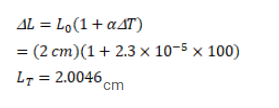# A circular hole of diameter 2.00 cm is made in an aluminium,Question:

A circular hole of diameter $2.00 \mathrm{~cm}$ is made in an aluminium, plate at $0^{\circ}$

C. What will be the diameter at $100^{\circ} \mathrm{C}$ ? a for aluminium $=2.3^{\times 10^{-5} /{ }^{\circ} \mathrm{C}}$

Solution: